# Ace or king

What is the probability that we will choose an ace or a king when choosing from a deck of sevens cards?

p =  0.25

### Step-by-step explanation: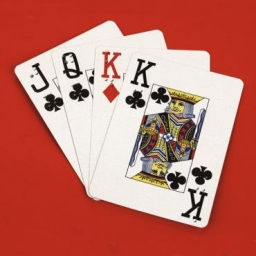Did you find an error or inaccuracy? Feel free to write us. Thank you!Tips to related online calculators
Would you like to compute count of combinations?

## Related math problems and questions:

• AceFrom complete sets of playing cards (32 cards), we pulled out one card. What is the probability of pulling the ace?
• CardsFrom a set of 32 cards, we randomly pull out three cards. What is the probability that it will be seven kings and ace?
• Hearts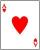5 cards are chosen from a standard deck of 52 playing cards (13 hearts) with replacement. What is the probability of choosing 5 hearts in a row?
• Three dice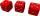What is the probability that when we roll three dice, we roll the numbers 1,2,3?
• Playing cards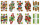From 32 playing cards containing 8 red cards, we choose 4 cards. What is the probability that just 2 will be red?
• DicesWe will throw two dice. What is the probability that the ratio between numbers on first and second dice will be 1:2?
• CardsThe player gets 8 cards of 32. What is the probability that it gets a) all 4 aces b) at least 1 ace
• Drawing from a hatWhen drawing numbers from a hat from 1 to 35, we select random given numbers. What is the probability that the drawn numbers will be divisible by 8 and 2?
• Sum 10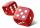What is the probability that two dice throw twice in a row will result the sum of 10?
• Balls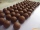From the bag with numbered balls (numbers 1,2,3,. ..20) we pick one ball. What is the probability of choosing a number containing 1?
• Event probability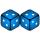The probability of event P in 8 independent experiments is 0.33. What is the probability that the event P occurs in one experiment (chance is the same)?
• The diceWhat is the probability of events that if we throw a dice is rolled less than 6?
• Three reds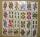What is the probability that when choosing 3 carats from seven carats, all 3 reds will be red?
• One threeWe throw two dice. What is the probability that max one three falls?
• ProbabilityHow probable is a randomly selected three-digit number divisible by five or seven?
• Two acesFrom a 32 card box we randomly pick 1 card and then 2 more cards. What is the probability that last two drawn cards are aces?
• Prime numberJan wrote any number from 1 to 20. What is the probability that he wrote the prime number?